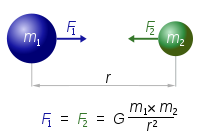## Newton's Law of universal gravitation Calculator

 Universal Gravitational Constant(G) = 6.6726 x 10-11N-m2/kg2 I want to calculate Gravitational Force (F) Mass of Object 1(m1) Mass of Object 2(m2) Distance Between the Objects (r) Mass of Object 1(m1) = kg Mass of Object 2(m2) = kg Distance Between the Objects (r) = m Gravitational Force (F) = N

Newton's law of universal gravitation is two bodies universe attraction between each other.

The Fig below show the formula and the attraction force F.### Newton's Law of Gravity formula:

Gravitational Force: F = Gm1m2/r2

where, m2 is Mass of Object 2, m1 is Mass of Object 1, G is Universal Gravitational Constant equals to 6.6726 x 10-11N-m2/kg2, r is Distance Between the Objects.

For example, when m1 = 2000000000KG, m2 = 5000000000KG, Distance = 600000m, Then Gravitational Force (F) = 185350000E-11.

Thinkcalculator.com provides you helpful and handy calculator resources.